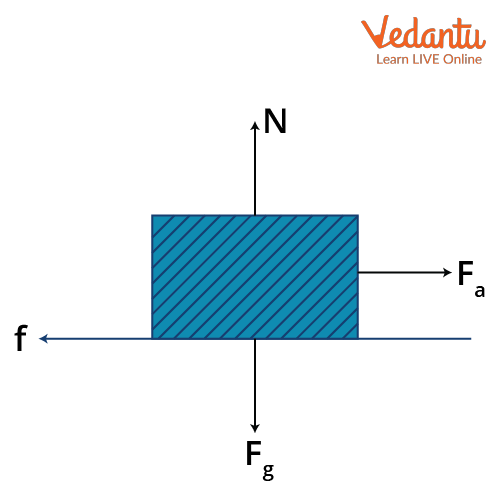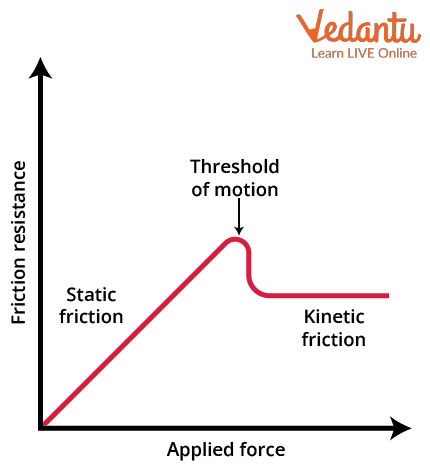Courses
Courses for Kids
Free study material
Free LIVE classes
More

# Static and Kinetic Friction - Important Concepts with Solved Examples for JEELIVE
Join Vedantu’s FREE Mastercalss

## Insights into Friction

Friction is a force which plays a major role in a human's life. Friction could be described as a force which opposes the motion of objects that are in contact with each other. The frictional force helps a human to walk on the road safely instead of rolling on the ground. The frictional force is denoted by the letter f and is measured in Newtons.

A material exhibits frictional force, which varies from object to object. This is measured with the help of the coefficient of friction. The coefficient of friction could be defined as the measure of friction present between two surfaces which are in contact. This is denoted by the symbol 𝜇 and it has no dimensions. It is defined by the formula $f = \mu N$ where N is the normal force acting upwards on the object.

The frictional force is divided into two types based on the movement of objects which are in contact with each other. These are static friction and kinetic friction. Static friction is a type of friction which opposes the motion of an object that is at rest. For example: when you’re trying to move a rock which is at rest, a force is experienced which opposes the movement of the rock. This is static friction.

Kinetic friction is the friction that opposes the movement between the moving surfaces. For example: a block experiences a force which opposes its motion on a surface. Let us get deeper into the concept of static and kinetic friction.

## Static and Kinetic Friction

### Static Friction

Let us assume an object is lying on a surface and is at rest. If we apply a force on it, there are four kinds of forces that act along with the applied force on the object. Those are the force of gravity in the download direction, the normal force acting on the object in the upward direction, the applied force acting in one direction and the frictional force acting in the direction opposite to the applied force as shown in the figure.Forces Acting on an Object

In this figure, Fg is the gravitational force, Fa is the applied force, N is the normal force and f is the friction. When the object is at rest and we are applying a force on it, we will be experiencing a small opposing force which resists the movement in the object. This is termed static friction.

In this type of friction, the frictional force always balances the applied force in order to keep the object at rest.

I.e., Fa = f as long as the object remains at rest.

This friction remains until the body starts moving with the force applied to it.

The static friction formula is defined as:

$f_{s}=\mu_{s} N$

where $\mu_{s}$ is the coefficient of static friction.

The static friction is independent of the surface area of contact and is directly proportional to the normal force acting on the object.

## Kinetic Friction

Similarly, kinetic friction is a kind of friction which opposes the motion of moving objects. If an object starts moving due to the applied force on it, then there is a frictional force which opposes the applied force of the object. This is kinetic friction. The kinetic friction can be found through the formula:

$f_{k}=\mu_{k} N$
where $\mu_{k}$ is the coefficient of kinetic friction.

The kinetic friction is also directly proportional to the normal force acting on the moving object and does not depend on the area of contact between two objects. If an object is applied with some force, the static friction resists it up to some point known as the threshold point after which the static friction starts decreasing. This results in the movement of the object. Then, the kinetic friction starts acting on the object. This is represented in the below graph of static and kinetic friction.Static and Kinetic Friction Graph

We learnt what is static friction and what is kinetic friction till now. Let us see some applications of static friction and kinetic friction.

## Applications of Static and Kinetic Friction

### Static Friction

• A car parked on a road.

• Block placed on a bench.

• A book lying on a table.

• Hook hanged to the wall.

• A photo frame on the wall.

### Kinetic Friction

• Producing fire through the rubbing of two stones.

• Chains and lubricant parts in machines.

• A moving car on a road.

• Skating on a skateboard.

• Playing slide in a park.

These are the applications of friction that we confront in our daily life. Let us see some examples solved in this concept.

## Solved Examples of Friction

Example 1: A book of mass 0.4kg is at rest on a table whose coefficient of static friction is 0.5. Find the static friction acting on the book.

Solution: Given: $\mu_{s} = 0.5$ and m = 0.4kg

The force of gravity: Fg = mg = 0.4 $\times$ 10 = 4N

When the object is at rest, the normal force is equal to the gravity force on the object.

N = Fg

Normal Force = 4N

We know that static friction: $f_{s}=\mu_{s} N$

I.e., Fs = (0.5)(4) = 2N

Hence, the static friction on the book is 2 newtons.

Example 2: The kinetic friction of an object moving on a surface is 15N where the mass of the object is 5kg. If the coefficient of kinetic friction is 0.7, then what is the horizontally applied force needed for the object to have a constant speed in its motion?

Solution: Given: $\mu_{k} = 0.7$ and m = 5kg

At constant speed, the object will be in equilibrium.

I.e., N = mg

Fa = fk

N = 5(10) = 50N

$f_{k}=\mu_{k} N$ = (0.7)50 = 35N

Therefore, the applied force is equal to the kinetic friction which is equal to 35N.

## Conclusion

Friction is a concept that plays a very important role in the motion of objects. We discussed what is static friction, what is kinetic friction and the formula for coefficient of static friction and kinetic friction. Static friction and kinetic friction are directly proportional to the normal force acting on the object in the upward direction and those are independent of the area of the surface of contact between the objects. We also derive kinetic friction and static friction formulas with valid explanations in this article.

Last updated date: 19th Sep 2023
Total views: 134.1k
Views today: 4.34k

## FAQs on Static and Kinetic Friction - Important Concepts with Solved Examples for JEE

1. Explain the distinction between static and kinetic friction?

The main difference between static and kinetic friction is that static friction is the friction which acts on the objects that are at rest, whereas, kinetic friction opposes the movement of objects which are in motion. Static friction has a maximum value called limited friction and when the applied force crosses the limited friction, the object will move, whereas, kinetic friction will be having a constant magnitude respective to the motion of the object related to the surface. The kinetic friction coefficient is smaller than the coefficient of static friction.

2. What is the weightage of this topic in JEE Main?

The topic of static friction and kinetic friction is very important in the JEE Mains examination. Learning about the coefficient of static friction and the coefficient of kinetic friction along with solving problems of the static friction formula is essential to scoring good marks in the JEE Mains. It has a weightage of 3.33% and a minimum of one question will be asked in the JEE Mains. Knowing what is the difference between static and kinetic friction is a basic foundation for the other topics in friction.